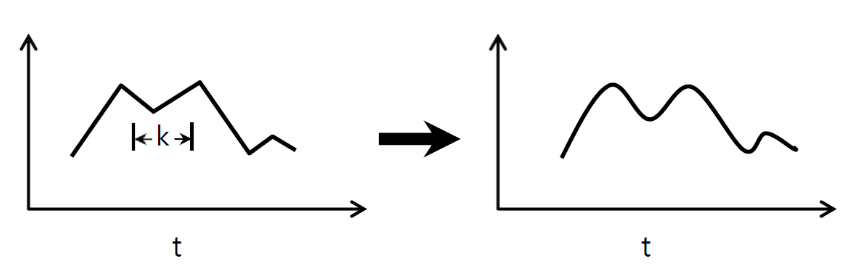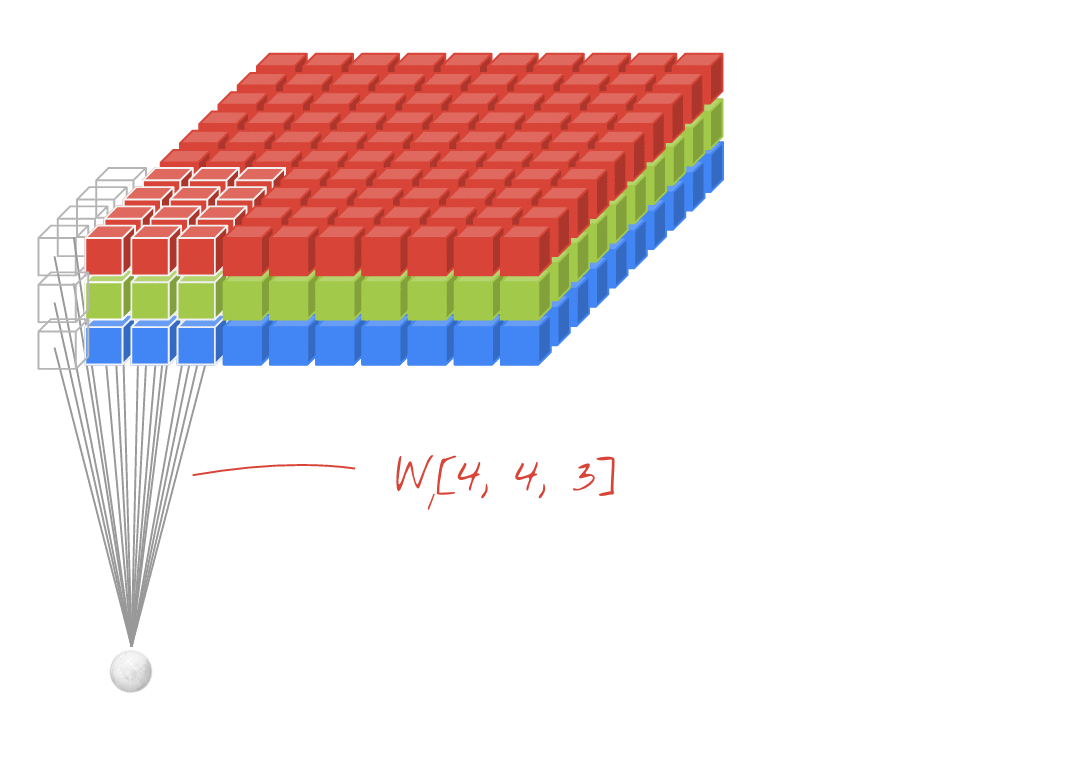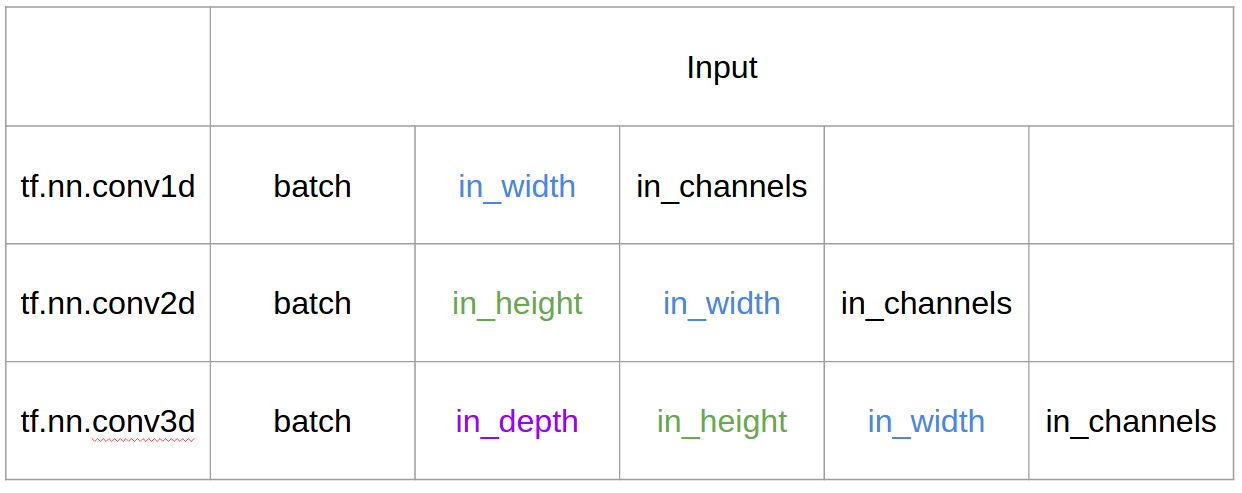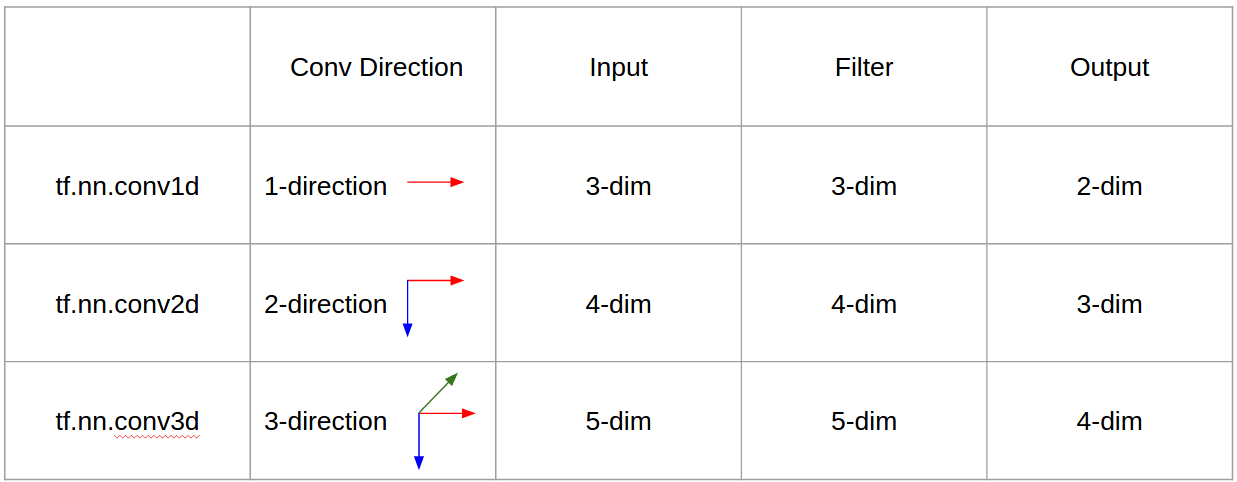# 1d 2d and 3d Convolution in CNN | CNN 1D vs 2D vs 3D

I want to explain with picture from C3D.

In a nutshell, convolutional direction & output shape is important!↑↑↑↑↑ 1D Convolutions – Basic ↑↑↑↑↑

• just 1-direction (time-axis) to calculate conv
• input = [W], filter = [k], output = [W]
• ex) input = [1,1,1,1,1], filter = [0.25,0.5,0.25], output = [1,1,1,1,1]
• output-shape is 1D array
• example) graph smoothing

### tf.nn.conv1d code Toy Example

``import tensorflow as tfimport numpy as npsess = tf.Session()ones_1d = np.ones(5)weight_1d = np.ones(3)strides_1d = 1in_1d = tf.constant(ones_1d, dtype=tf.float32)filter_1d = tf.constant(weight_1d, dtype=tf.float32)in_width = int(in_1d.shape)filter_width = int(filter_1d.shape)input_1d   = tf.reshape(in_1d, [1, in_width, 1])kernel_1d = tf.reshape(filter_1d, [filter_width, 1, 1])output_1d = tf.squeeze(tf.nn.conv1d(input_1d, kernel_1d, strides_1d, padding='SAME'))print sess.run(output_1d)``↑↑↑↑↑ 2D Convolutions – Basic ↑↑↑↑↑

• 2-direction (x,y) to calculate conv
• output-shape is 2D Matrix
• input = [W, H], filter = [k,k] output = [W,H]
• example) Sobel Egde Fllter

### tf.nn.conv2d – Toy Example

``ones_2d = np.ones((5,5))weight_2d = np.ones((3,3))strides_2d = [1, 1, 1, 1]in_2d = tf.constant(ones_2d, dtype=tf.float32)filter_2d = tf.constant(weight_2d, dtype=tf.float32)in_width = int(in_2d.shape)in_height = int(in_2d.shape)filter_width = int(filter_2d.shape)filter_height = int(filter_2d.shape)input_2d   = tf.reshape(in_2d, [1, in_height, in_width, 1])kernel_2d = tf.reshape(filter_2d, [filter_height, filter_width, 1, 1])output_2d = tf.squeeze(tf.nn.conv2d(input_2d, kernel_2d, strides=strides_2d, padding='SAME'))print sess.run(output_2d)``↑↑↑↑↑ 3D Convolutions – Basic ↑↑↑↑↑

• 3-direction (x,y,z) to calcuate conv
• output-shape is 3D Volume
• input = [W,H,L], filter = [k,k,d] output = [W,H,M]
• d < L is important! for making volume output
• example) C3D

### tf.nn.conv3d – Toy Example

``ones_3d = np.ones((5,5,5))weight_3d = np.ones((3,3,3))strides_3d = [1, 1, 1, 1, 1]in_3d = tf.constant(ones_3d, dtype=tf.float32)filter_3d = tf.constant(weight_3d, dtype=tf.float32)in_width = int(in_3d.shape)in_height = int(in_3d.shape)in_depth = int(in_3d.shape)filter_width = int(filter_3d.shape)filter_height = int(filter_3d.shape)filter_depth = int(filter_3d.shape)input_3d   = tf.reshape(in_3d, [1, in_depth, in_height, in_depth, 1])kernel_3d = tf.reshape(filter_3d, [filter_depth, filter_height, filter_width, 1, 1])output_3d = tf.squeeze(tf.nn.conv3d(input_3d, kernel_3d, strides=strides_3d, padding='SAME'))print sess.run(output_3d)``↑↑↑↑↑ 2D Convolutions with 3D input – LeNet, VGG, …, ↑↑↑↑↑

• Eventhough input is 3D ex) 224x224x3, 112x112x32
• output-shape is not 3D Volume, but 2D Matrix
• because filter depth = L must be matched with input channels = L
• 2-direction (x,y) to calcuate conv! not 3D
• input = [W,H,L], filter = [k,k,L] output = [W,H]
• output-shape is 2D Matrix
• what if we want to train N filters (N is number of filters)
• then output shape is (stacked 2D) 3D = 2D x N matrix.

### conv2d – LeNet, VGG, … for 1 filter

``in_channels = 32 # 3 for RGB, 32, 64, 128, ... ones_3d = np.ones((5,5,in_channels)) # input is 3d, in_channels = 32# filter must have 3d-shpae with in_channelsweight_3d = np.ones((3,3,in_channels)) strides_2d = [1, 1, 1, 1]in_3d = tf.constant(ones_3d, dtype=tf.float32)filter_3d = tf.constant(weight_3d, dtype=tf.float32)in_width = int(in_3d.shape)in_height = int(in_3d.shape)filter_width = int(filter_3d.shape)filter_height = int(filter_3d.shape)input_3d   = tf.reshape(in_3d, [1, in_height, in_width, in_channels])kernel_3d = tf.reshape(filter_3d, [filter_height, filter_width, in_channels, 1])output_2d = tf.squeeze(tf.nn.conv2d(input_3d, kernel_3d, strides=strides_2d, padding='SAME'))print sess.run(output_2d)``

### conv2d – LeNet, VGG, … for N filters

``in_channels = 32 # 3 for RGB, 32, 64, 128, ... out_channels = 64 # 128, 256, ...ones_3d = np.ones((5,5,in_channels)) # input is 3d, in_channels = 32# filter must have 3d-shpae x number of filters = 4Dweight_4d = np.ones((3,3,in_channels, out_channels))strides_2d = [1, 1, 1, 1]in_3d = tf.constant(ones_3d, dtype=tf.float32)filter_4d = tf.constant(weight_4d, dtype=tf.float32)in_width = int(in_3d.shape)in_height = int(in_3d.shape)filter_width = int(filter_4d.shape)filter_height = int(filter_4d.shape)input_3d   = tf.reshape(in_3d, [1, in_height, in_width, in_channels])kernel_4d = tf.reshape(filter_4d, [filter_height, filter_width, in_channels, out_channels])#output stacked shape is 3D = 2D x N matrixoutput_3d = tf.nn.conv2d(input_3d, kernel_4d, strides=strides_2d, padding='SAME')print sess.run(output_3d)``

↑↑↑↑↑ Bonus 1×1 conv in CNN – GoogLeNet, …, ↑↑↑↑↑

• 1×1 conv is confusing when you think this as 2D image filter like sobel
• for 1×1 conv in CNN, input is 3D shape as above picture.
• it calculate depth-wise filtering
• input = [W,H,L], filter = [1,1,L] output = [W,H]
• output stacked shape is 3D = 2D x N matrix.

### tf.nn.conv2d – special case 1×1 conv

``in_channels = 32 # 3 for RGB, 32, 64, 128, ... out_channels = 64 # 128, 256, ...ones_3d = np.ones((1,1,in_channels)) # input is 3d, in_channels = 32# filter must have 3d-shpae x number of filters = 4Dweight_4d = np.ones((3,3,in_channels, out_channels))strides_2d = [1, 1, 1, 1]in_3d = tf.constant(ones_3d, dtype=tf.float32)filter_4d = tf.constant(weight_4d, dtype=tf.float32)in_width = int(in_3d.shape)in_height = int(in_3d.shape)filter_width = int(filter_4d.shape)filter_height = int(filter_4d.shape)input_3d   = tf.reshape(in_3d, [1, in_height, in_width, in_channels])kernel_4d = tf.reshape(filter_4d, [filter_height, filter_width, in_channels, out_channels])#output stacked shape is 3D = 2D x N matrixoutput_3d = tf.nn.conv2d(input_3d, kernel_4d, strides=strides_2d, padding='SAME')print sess.run(output_3d)``

### Animation (2D Conv with 3D-inputs)– Original Link : LINK
– The author: Martin Görner

### Bonus 1D Convolutions with 2D input↑↑↑↑↑ 1D Convolutions with 1D input ↑↑↑↑↑↑↑↑↑↑ 1D Convolutions with 2D input ↑↑↑↑↑

• Eventhough input is 2D ex) 20×14
• output-shape is not 2D , but 1D Matrix
• because filter height = L must be matched with input height = L
• 1-direction (x) to calcuate conv! not 2D
• input = [W,L], filter = [k,L] output = [W]
• output-shape is 1D Matrix
• what if we want to train N filters (N is number of filters)
• then output shape is (stacked 1D) 2D = 1D x N matrix.

### Bonus C3D

``in_channels = 32 # 3, 32, 64, 128, ... out_channels = 64 # 3, 32, 64, 128, ... ones_4d = np.ones((5,5,5,in_channels))weight_5d = np.ones((3,3,3,in_channels,out_channels))strides_3d = [1, 1, 1, 1, 1]in_4d = tf.constant(ones_4d, dtype=tf.float32)filter_5d = tf.constant(weight_5d, dtype=tf.float32)in_width = int(in_4d.shape)in_height = int(in_4d.shape)in_depth = int(in_4d.shape)filter_width = int(filter_5d.shape)filter_height = int(filter_5d.shape)filter_depth = int(filter_5d.shape)input_4d   = tf.reshape(in_4d, [1, in_depth, in_height, in_depth, in_channels])kernel_5d = tf.reshape(filter_5d, [filter_depth, filter_height, filter_width, in_channels, out_channels])output_4d = tf.nn.conv3d(input_4d, kernel_5d, strides=strides_3d, padding='SAME')print sess.run(output_4d)sess.close()``

### Input & Output in Tensorflow### Summary